Publicité

# Solid State & Solution_Unit 1-2_Class-12_Board-JEE-NEET

9 May 2015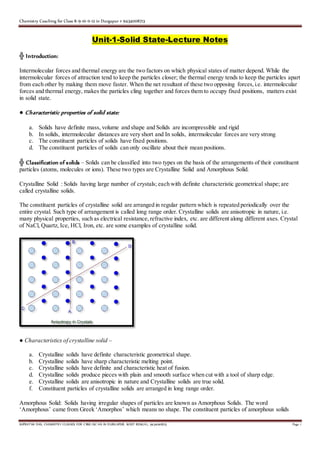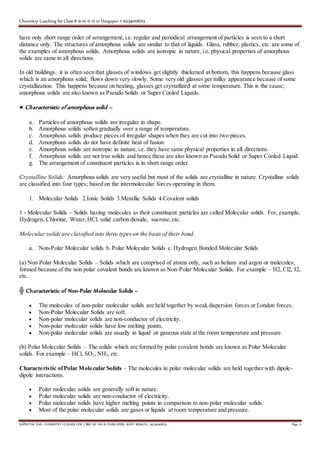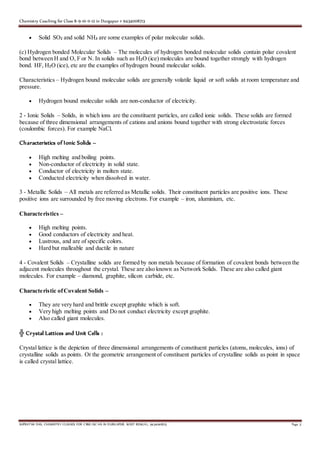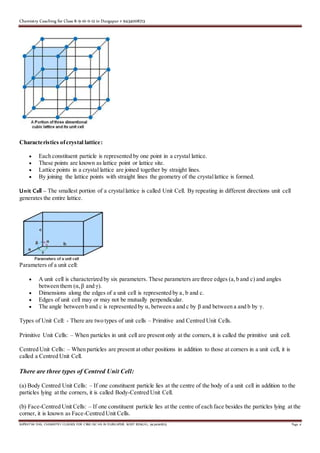Publicité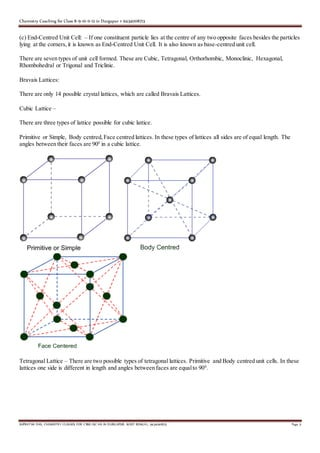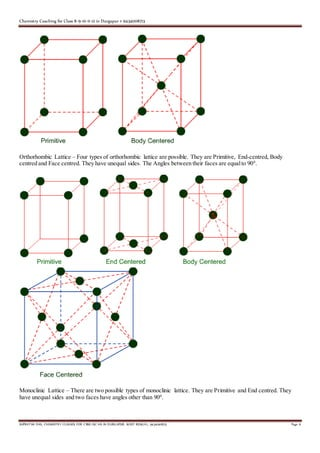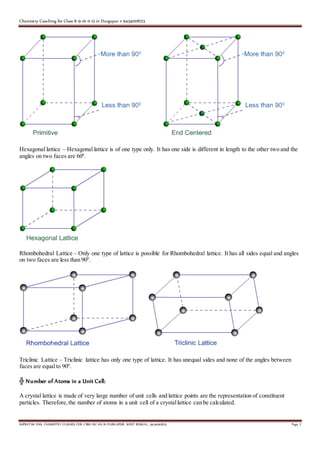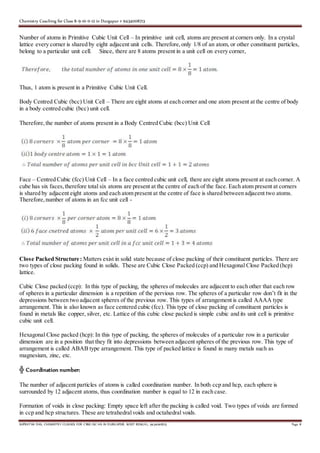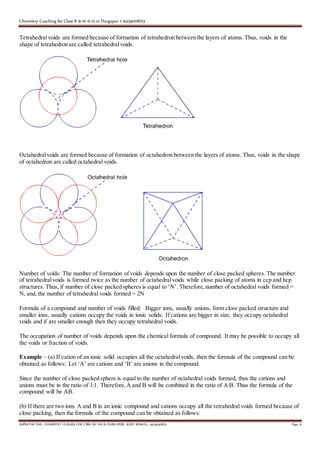Publicité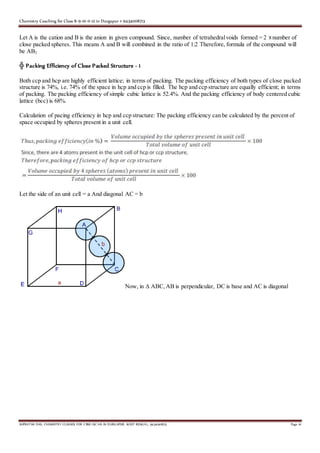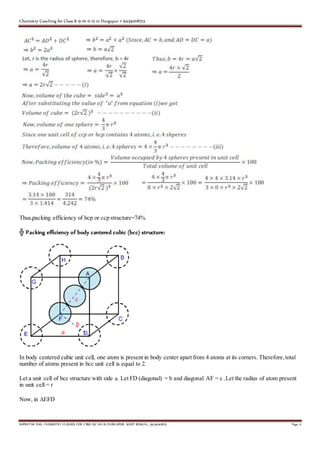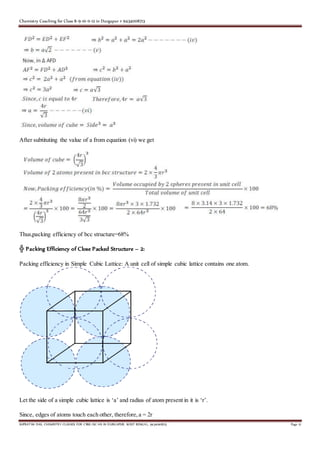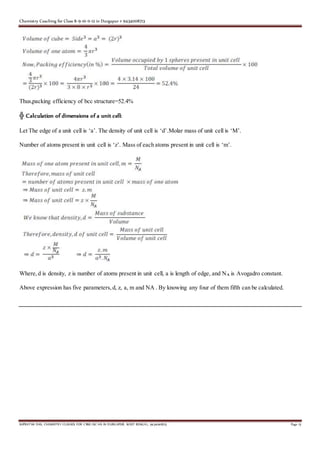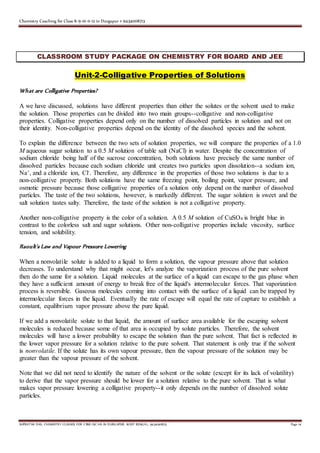Publicité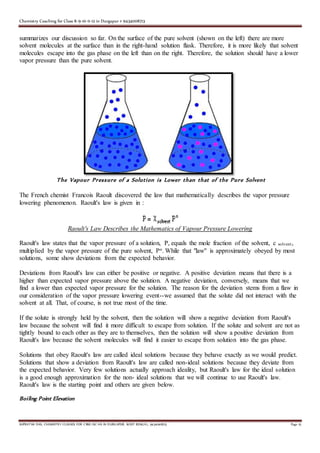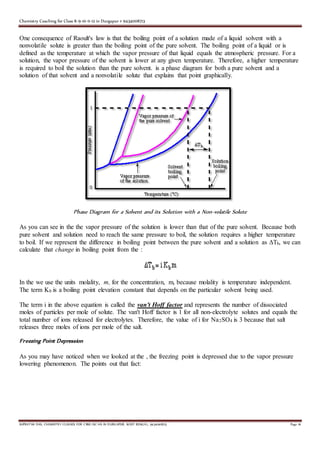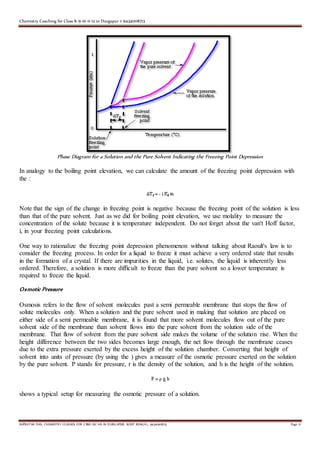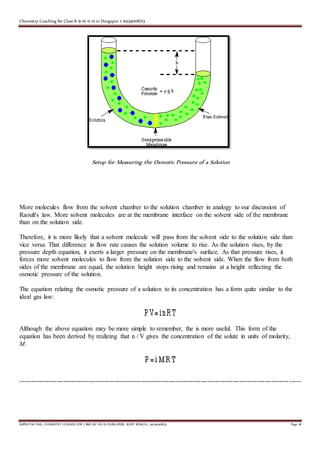Prochain SlideShareGseb class 12 chemistry sem 3 ch 1 solid state part 1
Chargement dans ... 3
1 sur 18
Publicité

### Solid State & Solution_Unit 1-2_Class-12_Board-JEE-NEET

1. Chemistry Coaching for Class 8-9-10-11-12 in Durgapur # 9434008713 SUPRATIM DAS, CHEMISTRY CLASSES FOR CBSE-ISC-HS IN D URGAPUR, WEST BENGAL, 9434008713 Page 1 Unit-1-Solid State-Lecture Notes ╬ Introduction: Intermolecular forces and thermal energy are the two factors on which physical states of matter depend. While the intermolecular forces of attraction tend to keep the particles closer; the thermal energy tends to keep the particles apart from each other by making them move faster. When the net resultant of these two opposing forces,i.e. intermolecular forces and thermal energy, makes the particles cling together and forces them to occupy fixed positions, matters exist in solid state. ● Characteristic properties of solid state: a. Solids have definite mass, volume and shape and Solids are incompressible and rigid b. In solids, intermolecular distances are very short and In solids, intermolecular forces are very strong c. The constituent particles of solids have fixed positions. d. The constituent particles of solids can only oscillate about their mean positions. ╬ Classification of solids – Solids can be classified into two types on the basis of the arrangements of their constituent particles (atoms, molecules or ions). These two types are Crystalline Solid and Amorphous Solid. Crystalline Solid : Solids having large number of crystals; each with definite characteristic geometrical shape; are called crystalline solids. The constituent particles of crystalline solid are arranged in regular pattern which is repeated periodically over the entire crystal. Such type of arrangement is called long range order. Crystalline solids are anisotropic in nature, i.e. many physical properties, such as electrical resistance,refractive index, etc. are different along different axes. Crystal of NaCl, Quartz, Ice, HCl, Iron, etc. are some examples of crystalline solid. ● Characteristics of crystalline solid – a. Crystalline solids have definite characteristic geometrical shape. b. Crystalline solids have sharp characteristic melting point. c. Crystalline solids have definite and characteristic heat of fusion. d. Crystalline solids produce pieces with plain and smooth surface when cut with a tool of sharp edge. e. Crystalline solids are anisotropic in nature and Crystalline solids are true solid. f. Constituent particles of crystalline solids are arranged in long range order. Amorphous Solid: Solids having irregular shapes of particles are known as Amorphous Solids. The word ‘Amorphous’ came from Greek ‘Amorphos’ which means no shape. The constituent particles of amorphous solids
2. Chemistry Coaching for Class 8-9-10-11-12 in Durgapur # 9434008713 SUPRATIM DAS, CHEMISTRY CLASSES FOR CBSE-ISC-HS IN D URGAPUR, WEST BENGAL, 9434008713 Page 2 have only short range order of arrangement, i.e. regular and periodical arrangement of particles is seen to a short distance only. The structures of amorphous solids are similar to that of liquids. Glass, rubber, plastics, etc. are some of the examples of amorphous solids. Amorphous solids are isotropic in nature, i.e. physical properties of amorphous solids are same in all directions. In old buildings, it is often seen that glasses of windows get slightly thickened at bottom, this happens because glass which is an amorphous solid; flows down very slowly. Some very old glasses get milky appearance because of some crystallization. This happens because on heating, glasses get crystallized at some temperature. This is the cause; amorphous solids are also known as Pseudo Solids or Super Cooled Liquids. ● Characteristic of amorphous solid – a. Particles of amorphous solids are irregular in shape. b. Amorphous solids soften gradually over a range of temperature. c. Amorphous solids produce pieces of irregular shapes when they are cut into two pieces. d. Amorphous solids do not have definite heat of fusion. e. Amorphous solids are isotropic in nature, i.e. they have same physical properties in all directions. f. Amorphous solids are not true solids and hence these are also known as Pseudo Solid or Super Cooled Liquid. g. The arrangement of constituent particles is in short range order. Crystalline Solids: Amorphous solids are very useful but most of the solids are crystalline in nature. Crystalline solids are classified into four types; based on the intermolecular forces operating in them. 1. Molecular Solids 2.Ionic Solids 3.Metallic Solids 4.Covalent solids 1 - Molecular Solids – Solids having molecules as their constituent particles are called Molecular solids. For, example, Hydrogen, Chlorine, Water,HCl, solid carbon dioxide, sucrose,etc. Molecular solids are classified into three typeson the basis of their bond: a. Non-Polar Molecular solids b. Polar Molecular Solids c. Hydrogen Bonded Molecular Solids (a) Non Polar Molecular Solids – Solids which are comprised of atoms only, such as helium and argon or molecules; formed because of the non polar covalent bonds are known as Non-Polar Molecular Solids. For example – H2, Cl2, I2, etc. ╬ Characteristic of Non-Polar Molecular Solids –  The molecules of non-polar molecular solids are held together by weak dispersion forces or London forces.  Non-Polar Molecular Solids are soft.  Non-polar molecular solids are non-conductor of electricity.  Non-polar molecular solids have low melting points.  Non-polar molecular solids are usually in liquid or gaseous state at the room temperature and pressure. (b) Polar Molecular Solids – The solids which are formed by polar covalent bonds are known as Polar Molecular solids. For example – HCl, SO2, NH3,etc. Characteristic ofPolar Molecular Solids – The molecules in polar molecular solids are held together with dipole- dipole interactions.  Polar molecular solids are generally soft in nature.  Polar molecular solids are non-conductor of electricity.  Polar molecular solids have higher melting points in comparison to non-polar molecular solids.  Most of the polar molecular solids are gases or liquids at room temperature and pressure.
3. Chemistry Coaching for Class 8-9-10-11-12 in Durgapur # 9434008713 SUPRATIM DAS, CHEMISTRY CLASSES FOR CBSE-ISC-HS IN D URGAPUR, WEST BENGAL, 9434008713 Page 3  Solid SO2 and solid NH3 are some examples of polar molecular solids. (c) Hydrogen bonded Molecular Solids – The molecules of hydrogen bonded molecular solids contain polar covalent bond between H and O, F or N. In solids such as H2O (ice) molecules are bound together strongly with hydrogen bond. HF, H2O (ice), etc are the examples of hydrogen bound molecular solids. Characteristics – Hydrogen bound molecular solids are generally volatile liquid or soft solids at room temperature and pressure.  Hydrogen bound molecular solids are non-conductor of electricity. 2 - Ionic Solids – Solids, in which ions are the constituent particles, are called ionic solids. These solids are formed because of three dimensional arrangements of cations and anions bound together with strong electrostatic forces (coulombic forces). For example NaCl. Characteristics of Ionic Solids –  High melting and boiling points.  Non-conductor of electricity in solid state.  Conductor of electricity in molten state.  Conducted electricity when dissolved in water. 3 - Metallic Solids – All metals are referred as Metallic solids. Their constituent particles are positive ions. These positive ions are surrounded by free moving electrons. For example – iron, aluminium, etc. Characteristics –  High melting points.  Good conductors of electricity and heat.  Lustrous, and are of specific colors.  Hard but malleable and ductile in nature 4 - Covalent Solids – Crystalline solids are formed by non metals because of formation of covalent bonds between the adjacent molecules throughout the crystal. These are also known as Network Solids. These are also called giant molecules. For example – diamond, graphite, silicon carbide, etc. Characteristic ofCovalent Solids –  They are very hard and brittle except graphite which is soft.  Very high melting points and Do not conduct electricity except graphite.  Also called giant molecules. ╬ Crystal Lattices and Unit Cells : Crystal lattice is the depiction of three dimensional arrangements of constituent particles (atoms, molecules, ions) of crystalline solids as points. Or the geometric arrangement of constituent particles of crystalline solids as point in space is called crystal lattice.
4. Chemistry Coaching for Class 8-9-10-11-12 in Durgapur # 9434008713 SUPRATIM DAS, CHEMISTRY CLASSES FOR CBSE-ISC-HS IN D URGAPUR, WEST BENGAL, 9434008713 Page 4 Characteristics ofcrystal lattice:  Each constituent particle is represented by one point in a crystal lattice.  These points are known as lattice point or lattice site.  Lattice points in a crystal lattice are joined together by straight lines.  By joining the lattice points with straight lines the geometry of the crystallattice is formed. Unit Cell – The smallest portion of a crystallattice is called Unit Cell. By repeating in different directions unit cell generates the entire lattice. Parameters of a unit cell:  A unit cell is characterized by six parameters. These parameters are three edges (a,b and c) and angles between them (α,β and γ).  Dimensions along the edges of a unit cell is represented by a, b and c.  Edges of unit cell may or may not be mutually perpendicular.  The angle between b and c is represented by α, between a and c by β and between a and b by γ. Types of Unit Cell: - There are two types of unit cells – Primitive and Centred Unit Cells. Primitive Unit Cells: – When particles in unit cell are present only at the corners,it is called the primitive unit cell. Centred Unit Cells: – When particles are present at other positions in addition to those at corners in a unit cell, it is called a Centred Unit Cell. There are three types of Centred Unit Cell: (a) Body Centred Unit Cells: – If one constituent particle lies at the centre of the body of a unit cell in addition to the particles lying at the corners, it is called Body-Centred Unit Cell. (b) Face-Centred Unit Cells: – If one constituent particle lies at the centre of each face besides the particles lying at the corner, it is known as Face-Centred Unit Cells.
5. Chemistry Coaching for Class 8-9-10-11-12 in Durgapur # 9434008713 SUPRATIM DAS, CHEMISTRY CLASSES FOR CBSE-ISC-HS IN D URGAPUR, WEST BENGAL, 9434008713 Page 5 (c) End-Centred Unit Cell: – If one constituent particle lies at the centre of any two opposite faces besides the particles lying at the corners,it is known as End-Centred Unit Cell. It is also known as base-centred unit cell. There are seven types of unit cell formed. These are Cubic, Tetragonal, Orthorhombic, Monoclinic, Hexagonal, Rhombohedral or Trigonal and Triclinic. Bravais Lattices: There are only 14 possible crystal lattices, which are called Bravais Lattices. Cubic Lattice – There are three types of lattice possible for cubic lattice. Primitive or Simple, Body centred,Face centred lattices. In these types of lattices all sides are of equal length. The angles between their faces are 900 in a cubic lattice. Tetragonal Lattice – There are two possible types of tetragonal lattices. Primitive and Body centred unit cells. In these lattices one side is different in length and angles between faces are equalto 900 .
6. Chemistry Coaching for Class 8-9-10-11-12 in Durgapur # 9434008713 SUPRATIM DAS, CHEMISTRY CLASSES FOR CBSE-ISC-HS IN D URGAPUR, WEST BENGAL, 9434008713 Page 6 Orthorhombic Lattice – Four types of orthorhombic lattice are possible. They are Primitive, End-centred, Body centred and Face centred. They have unequal sides. The Angles between their faces are equalto 900 . Monoclinic Lattice – There are two possible types of monoclinic lattice. They are Primitive and End centred. They have unequal sides and two faces have angles other than 900 .
7. Chemistry Coaching for Class 8-9-10-11-12 in Durgapur # 9434008713 SUPRATIM DAS, CHEMISTRY CLASSES FOR CBSE-ISC-HS IN D URGAPUR, WEST BENGAL, 9434008713 Page 7 Hexagonal lattice – Hexagonal lattice is of one type only. It has one side is different in length to the other two and the angles on two faces are 600 . Rhombohedral Lattice – Only one type of lattice is possible for Rhombohedral lattice. It has all sides equal and angles on two faces are less than 900 . Triclinic Lattice – Triclinic lattice has only one type of lattice. It has unequal sides and none of the angles between faces are equalto 900 . ╬ Number of Atoms in a Unit Cell: A crystal lattice is made of very large number of unit cells and lattice points are the representation of constituent particles. Therefore, the number of atoms in a unit cell of a crystallattice can be calculated.
8. Chemistry Coaching for Class 8-9-10-11-12 in Durgapur # 9434008713 SUPRATIM DAS, CHEMISTRY CLASSES FOR CBSE-ISC-HS IN D URGAPUR, WEST BENGAL, 9434008713 Page 8 Number of atoms in Primitive Cubic Unit Cell – In primitive unit cell, atoms are present at corners only. In a crystal lattice every corner is shared by eight adjacent unit cells. Therefore,only 1/8 of an atom, or other constituent particles, belong to a particular unit cell. Since, there are 8 atoms present in a unit cell on every corner, Thus, 1 atom is present in a Primitive Cubic Unit Cell. Body Centred Cubic (bcc) Unit Cell – There are eight atoms at each corner and one atom present at the centre of body in a body centred cubic (bcc) unit cell. Therefore,the number of atoms present in a Body Centred Cubic (bcc) Unit Cell Face – Centred Cubic (fcc) Unit Cell – In a face centred cubic unit cell, there are eight atoms present at each corner. A cube has six faces,therefore total six atoms are present at the centre of each of the face. Each atom present at corners is shared by adjacent eight atoms and each atom present at the centre of face is shared between adjacent two atoms. Therefore,number of atoms in an fcc unit cell - Close Packed Structure: Matters exist in solid state because of close packing of their constituent particles. There are two types of close packing found in solids. These are Cubic Close Packed (ccp) and Hexagonal Close Packed (hcp) lattice. Cubic Close packed (ccp): In this type of packing, the spheres of molecules are adjacent to each other that each row of spheres in a particular dimension is a repetition of the pervious row. The spheres of a particular row don’t fit in the depressions between two adjacent spheres of the previous row. This types of arrangement is called AAAA type arrangement. This is also known as face centered cubic (fcc). This type of close packing of constituent particles is found in metals like copper, silver, etc. Lattice of this cubic close packed is simple cubic and its unit cell is primitive cubic unit cell. Hexagonal Close packed (hcp): In this type of packing, the spheres of molecules of a particular row in a particular dimension are in a position that they fit into depressions between adjacent spheres of the previous row. This type of arrangement is called ABAB type arrangement. This type of packed lattice is found in many metals such as magnesium, zinc, etc. ╬ Coordination number: The number of adjacent particles of atoms is called coordination number. In both ccp and hcp, each sphere is surrounded by 12 adjacent atoms, thus coordination number is equal to 12 in each case. Formation of voids in close packing: Empty space left after the packing is called void. Two types of voids are formed in ccp and hcp structures. These are tetrahedralvoids and octahedral voids.
9. Chemistry Coaching for Class 8-9-10-11-12 in Durgapur # 9434008713 SUPRATIM DAS, CHEMISTRY CLASSES FOR CBSE-ISC-HS IN D URGAPUR, WEST BENGAL, 9434008713 Page 9 Tetrahedralvoids are formed because of formation of tetrahedron between the layers of atoms. Thus, voids in the shape of tetrahedron are called tetrahedralvoids. Octahedralvoids are formed because of formation of octahedron between the layers of atoms. Thus, voids in the shape of octahedron are called octahedral voids. Number of voids: The number of formation of voids depends upon the number of close packed spheres. The number of tetrahedralvoids is formed twice as the number of octahedralvoids while close packing of atoms in ccp and hcp structures. Thus, if number of close packed spheres is equal to ‘N’. Therefore,number of octahedral voids formed = N, and, the number of tetrahedral voids formed = 2N Formula of a compound and number of voids filled: Bigger ions, usually anions, form close packed structure and smaller ions, usually cations occupy the voids in ionic solids. If cations are bigger in size, they occupy octahedral voids and if are smaller enough then they occupy tetrahedral voids. The occupation of number of voids depends upon the chemical formula of compound. It may be possible to occupy all the voids or fraction of voids. Example – (a) If cation of an ionic solid occupies all the octahedralvoids, then the formula of the compound can be obtained as follows: Let ‘A’ are cations and ‘B’ are anions in the compound. Since the number of close packed sphere is equal to the number of octahedral voids formed, thus the cations and anions must be in the ratio of 1:1. Therefore,A and B will be combined in the ratio of A:B. Thus the formula of the compound will be AB. (b) If there are two ions A and B in an ionic compound and cations occupy all the tetrahedral voids formed because of close packing, then the formula of the compound can be obtained as follows:
10. Chemistry Coaching for Class 8-9-10-11-12 in Durgapur # 9434008713 SUPRATIM DAS, CHEMISTRY CLASSES FOR CBSE-ISC-HS IN D URGAPUR, WEST BENGAL, 9434008713 Page 10 Let A is the cation and B is the anion in given compound. Since, number of tetrahedralvoids formed = 2 X number of close packed spheres. This means A and B will combined in the ratio of 1:2 Therefore, formula of the compound will be AB2 ╬ Packing Efficiency of Close Packed Structure - 1 Both ccp and hcp are highly efficient lattice; in terms of packing. The packing efficiency of both types of close packed structure is 74%, i.e. 74% of the space in hcp and ccp is filled. The hcp and ccp structure are equally efficient; in terms of packing. The packing efficiency of simple cubic lattice is 52.4%. And the packing efficiency of body centered cubic lattice (bcc) is 68%. Calculation of pacing efficiency in hcp and ccp structure: The packing efficiency can be calculated by the percent of space occupied by spheres present in a unit cell. Let the side of an unit cell = a And diagonal AC = b Now, in ∆ ABC,AB is perpendicular, DC is base and AC is diagonal
11. Chemistry Coaching for Class 8-9-10-11-12 in Durgapur # 9434008713 SUPRATIM DAS, CHEMISTRY CLASSES FOR CBSE-ISC-HS IN D URGAPUR, WEST BENGAL, 9434008713 Page 11 Thus,packing efficiency of hcp or ccp structure=74% ╬ Packing efficiency of body cantered cubic (bcc) structure: In body centered cubic unit cell, one atom is present in body center apart from 4 atoms at its corners. Therefore,total number of atoms present in bcc unit cell is equal to 2. Let a unit cell of bcc structure with side a. Let FD (diagonal) = b and diagonal AF = c .Let the radius of atom present in unit cell = r Now, in ∆EFD
12. Chemistry Coaching for Class 8-9-10-11-12 in Durgapur # 9434008713 SUPRATIM DAS, CHEMISTRY CLASSES FOR CBSE-ISC-HS IN D URGAPUR, WEST BENGAL, 9434008713 Page 12 After subtituting the value of a from equation (vi) we get Thus,packing efficiency of bcc structure=68% ╬ Packing Efficiency of Close Packed Structure – 2: Packing efficiency in Simple Cubic Lattice: A unit cell of simple cubic lattice contains one atom. Let the side of a simple cubic lattice is ‘a’ and radius of atom present in it is ‘r’. Since, edges of atoms touch each other, therefore,a = 2r
13. Chemistry Coaching for Class 8-9-10-11-12 in Durgapur # 9434008713 SUPRATIM DAS, CHEMISTRY CLASSES FOR CBSE-ISC-HS IN D URGAPUR, WEST BENGAL, 9434008713 Page 13 Thus,packing efficiency of bcc structure=52.4% ╬ Calculation of dimensions of a unit cell: Let The edge of a unit cell is ‘a’. The density of unit cell is ‘d’.Molar mass of unit cell is ‘M’. Number of atoms present in unit cell is ‘z’. Mass of each atoms present in unit cell is ‘m’. Where,d is density, z is number of atoms present in unit cell, a is length of edge, and NA is Avogadro constant. Above expression has five parameters,d, z, a, m and NA . By knowing any four of them fifth can be calculated.
14. Chemistry Coaching for Class 8-9-10-11-12 in Durgapur # 9434008713 SUPRATIM DAS, CHEMISTRY CLASSES FOR CBSE-ISC-HS IN D URGAPUR, WEST BENGAL, 9434008713 Page 14 CLASSROOM STUDY PACKAGE ON CHEMISTRY FOR BOARD AND JEE Unit-2-Colligative Properties of Solutions What are Colligative Properties? A we have discussed, solutions have different properties than either the solutes or the solvent used to make the solution. Those properties can be divided into two main groups--colligative and non-colligative properties. Colligative properties depend only on the number of dissolved particles in solution and not on their identity. Non-colligative properties depend on the identity of the dissolved species and the solvent. To explain the difference between the two sets of solution properties, we will compare the properties of a 1.0 M aqueous sugar solution to a 0.5 M solution of table salt (NaCl) in water. Despite the concentration of sodium chloride being half of the sucrose concentration, both solutions have precisely the same number of dissolved particles because each sodium chloride unit creates two particles upon dissolution--a sodium ion, Na+, and a chloride ion, Cl-. Therefore, any difference in the properties of those two solutions is due to a non-colligative property. Both solutions have the same freezing point, boiling point, vapor pressure, and osmotic pressure because those colligative properties of a solution only depend on the number of dissolved particles. The taste of the two solutions, however, is markedly different. The sugar solution is sweet and the salt solution tastes salty. Therefore, the taste of the solution is not a colligative property. Another non-colligative property is the color of a solution. A 0.5 M solution of CuSO4 is bright blue in contrast to the colorless salt and sugar solutions. Other non-colligative properties include viscosity, surface tension, and solubility. Raoult's Law and Vapour Pressure Lowering When a nonvolatile solute is added to a liquid to form a solution, the vapour pressure above that solution decreases. To understand why that might occur, let's analyze the vaporization process of the pure solvent then do the same for a solution. Liquid molecules at the surface of a liquid can escape to the gas phase when they have a sufficient amount of energy to break free of the liquid's intermolecular forces. That vaporization process is reversible. Gaseous molecules coming into contact with the surface of a liquid can be trapped by intermolecular forces in the liquid. Eventually the rate of escape will equal the rate of capture to establish a constant, equilibrium vapor pressure above the pure liquid. If we add a nonvolatile solute to that liquid, the amount of surface area available for the escaping solvent molecules is reduced because some of that area is occupied by solute particles. Therefore, the solvent molecules will have a lower probability to escape the solution than the pure solvent. That fact is reflected in the lower vapor pressure for a solution relative to the pure solvent. That statement is only true if the solvent is nonvolatile. If the solute has its own vapour pressure, then the vapour pressure of the solution may be greater than the vapour pressure of the solvent. Note that we did not need to identify the nature of the solvent or the solute (except for its lack of volatility) to derive that the vapor pressure should be lower for a solution relative to the pure solvent. That is what makes vapor pressure lowering a colligative property--it only depends on the number of dissolved solute particles.
15. Chemistry Coaching for Class 8-9-10-11-12 in Durgapur # 9434008713 SUPRATIM DAS, CHEMISTRY CLASSES FOR CBSE-ISC-HS IN D URGAPUR, WEST BENGAL, 9434008713 Page 15 summarizes our discussion so far. On the surface of the pure solvent (shown on the left) there are more solvent molecules at the surface than in the right-hand solution flask. Therefore, it is more likely that solvent molecules escape into the gas phase on the left than on the right. Therefore, the solution should have a lower vapor pressure than the pure solvent. The Vapour Pressure of a Solution is Lower than that of the Pure Solvent The French chemist Francois Raoult discovered the law that mathematically describes the vapor pressure lowering phenomenon. Raoult's law is given in : Raoult's Law Describes the Mathematics of Vapour Pressure Lowering Raoult's law states that the vapor pressure of a solution, P, equals the mole fraction of the solvent, c solvent, multiplied by the vapor pressure of the pure solvent, Po. While that "law" is approximately obeyed by most solutions, some show deviations from the expected behavior. Deviations from Raoult's law can either be positive or negative. A positive deviation means that there is a higher than expected vapor pressure above the solution. A negative deviation, conversely, means that we find a lower than expected vapor pressure for the solution. The reason for the deviation stems from a flaw in our consideration of the vapor pressure lowering event--we assumed that the solute did not interact with the solvent at all. That, of course, is not true most of the time. If the solute is strongly held by the solvent, then the solution will show a negative deviation from Raoult's law because the solvent will find it more difficult to escape from solution. If the solute and solvent are not as tightly bound to each other as they are to themselves, then the solution will show a positive deviation from Raoult's law because the solvent molecules will find it easier to escape from solution into the gas phase. Solutions that obey Raoult's law are called ideal solutions because they behave exactly as we would predict. Solutions that show a deviation from Raoult's law are called non-ideal solutions because they deviate from the expected behavior. Very few solutions actually approach ideality, but Raoult's law for the ideal solution is a good enough approximation for the non- ideal solutions that we will continue to use Raoult's law. Raoult's law is the starting point and others are given below. Boiling Point Elevation
16. Chemistry Coaching for Class 8-9-10-11-12 in Durgapur # 9434008713 SUPRATIM DAS, CHEMISTRY CLASSES FOR CBSE-ISC-HS IN D URGAPUR, WEST BENGAL, 9434008713 Page 16 One consequence of Raoult's law is that the boiling point of a solution made of a liquid solvent with a nonvolatile solute is greater than the boiling point of the pure solvent. The boiling point of a liquid or is defined as the temperature at which the vapor pressure of that liquid equals the atmospheric pressure. For a solution, the vapor pressure of the solvent is lower at any given temperature. Therefore, a higher temperature is required to boil the solution than the pure solvent. is a phase diagram for both a pure solvent and a solution of that solvent and a nonvolatile solute that explains that point graphically. Phase Diagram for a Solvent and its Solution with a Non-volatile Solute As you can see in the the vapor pressure of the solution is lower than that of the pure solvent. Because both pure solvent and solution need to reach the same pressure to boil, the solution requires a higher temperature to boil. If we represent the difference in boiling point between the pure solvent and a solution as ΔTb, we can calculate that change in boiling point from the : In the we use the units molality, m, for the concentration, m, because molality is temperature independent. The term Kb is a boiling point elevation constant that depends on the particular solvent being used. The term i in the above equation is called the van't Hoff factor and represents the number of dissociated moles of particles per mole of solute. The van't Hoff factor is 1 for all non-electrolyte solutes and equals the total number of ions released for electrolytes. Therefore, the value of i for Na2SO4 is 3 because that salt releases three moles of ions per mole of the salt. Freezing Point Depression As you may have noticed when we looked at the , the freezing point is depressed due to the vapor pressure lowering phenomenon. The points out that fact:
17. Chemistry Coaching for Class 8-9-10-11-12 in Durgapur # 9434008713 SUPRATIM DAS, CHEMISTRY CLASSES FOR CBSE-ISC-HS IN D URGAPUR, WEST BENGAL, 9434008713 Page 17 Phase Diagram for a Solution and the Pure Solvent Indicating the Freezing Point Depression In analogy to the boiling point elevation, we can calculate the amount of the freezing point depression with the : Note that the sign of the change in freezing point is negative because the freezing point of the solution is less than that of the pure solvent. Just as we did for boiling point elevation, we use molality to measure the concentration of the solute because it is temperature independent. Do not forget about the van't Hoff factor, i, in your freezing point calculations. One way to rationalize the freezing point depression phenomenon without talking about Raoult's law is to consider the freezing process. In order for a liquid to freeze it must achieve a very ordered state that results in the formation of a crystal. If there are impurities in the liquid, i.e. solutes, the liquid is inherently less ordered. Therefore, a solution is more difficult to freeze than the pure solvent so a lower temperature is required to freeze the liquid. Osmotic Pressure Osmosis refers to the flow of solvent molecules past a semi permeable membrane that stops the flow of solute molecules only. When a solution and the pure solvent used in making that solution are placed on either side of a semi permeable membrane, it is found that more solvent molecules flow out of the pure solvent side of the membrane than solvent flows into the pure solvent from the solution side of the membrane. That flow of solvent from the pure solvent side makes the volume of the solution rise. When the height difference between the two sides becomes large enough, the net flow through the membrane ceases due to the extra pressure exerted by the excess height of the solution chamber. Converting that height of solvent into units of pressure (by using the ) gives a measure of the osmotic pressure exerted on the solution by the pure solvent. P stands for pressure, r is the density of the solution, and h is the height of the solution. shows a typical setup for measuring the osmotic pressure of a solution.
18. Chemistry Coaching for Class 8-9-10-11-12 in Durgapur # 9434008713 SUPRATIM DAS, CHEMISTRY CLASSES FOR CBSE-ISC-HS IN D URGAPUR, WEST BENGAL, 9434008713 Page 18 Setup for Measuring the Osmotic Pressure of a Solution More molecules flow from the solvent chamber to the solution chamber in analogy to our discussion of Raoult's law. More solvent molecules are at the membrane interface on the solvent side of the membrane than on the solution side. Therefore, it is more likely that a solvent molecule will pass from the solvent side to the solution side than vice versa. That difference in flow rate causes the solution volume to rise. As the solution rises, by the pressure depth equation, it exerts a larger pressure on the membrane's surface. As that pressure rises, it forces more solvent molecules to flow from the solution side to the solvent side. When the flow from both sides of the membrane are equal, the solution height stops rising and remains at a height reflecting the osmotic pressure of the solution. The equation relating the osmotic pressure of a solution to its concentration has a form quite similar to the ideal gas law: Although the above equation may be more simple to remember, the is more useful. This form of the equation has been derived by realizing that n / V gives the concentration of the solute in units of molarity, M. ----------------------------------------------------------------------------------------------------------------------------------
Publicité